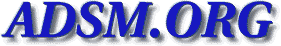Veritas-bu [Top] [All Lists]

## [Veritas-bu] Veritas Volume Replicator

2005-08-05 15:43:51
 Subject: [Veritas-bu] Veritas Volume Replicator Tammy.Yosifovski AT usfc DOT com (Yosifovski, Tammy) Fri, 5 Aug 2005 15:43:51 -0400
 ```This is a multi-part message in MIME format. ------_=_NextPart_001_01C599F6.0138E54E Content-Type: text/plain; charset="US-ASCII" Content-Transfer-Encoding: quoted-printable Does anyone use Veritas' Volume Replicator as part of their backup=0D=0Asol= ution=3F Thoughts on the product=3F Any suggestion on another=0D=0Areplic= ation product=3F=0D=0A=0D=0AThanks for your input and happy Friday.=0D=0A=0D= =0A=0D=0A=0D=0A------------------------------------------------------------= -------------------------=0D=0AThis e-mail is the exclusive, private and co= nfidential property of the sender. The=0D=0Ainformation contained in it is = intended solely for the use of the sender and the=0D=0Aintended recipient. = If you are not the intended recipient you are hereby advised that=0D=0Aany = unauthorized disclosure, copying, distribution or the taking of any action = in=0D=0Areliance on the contents of this message is strictly prohibited. If= you have received=0D=0Athis e-mail in error, please notify us immediately = by telephone (call the USF=0D=0ACorporation Technical Support Center at 1-8= 88-873-8721) and then destroy this=0D=0Adocument and any copies in any form= immediately. Finally, the recipient should=0D=0Acheck this email and any = attachments for the presence of viruses. USF Corporation=0D=0Aaccepts no li= ability for any damage caused by any virus transmitted by this email.=0D=0A= ---------------------------------------------------------------------------= ----------=0D=0A=0D=0A ------_=_NextPart_001_01C599F6.0138E54E Content-Type: text/html; charset="US-ASCII" Content-Transfer-Encoding: quoted-printable =0D=0A=0D=0A=0D= =0A=0D=0A=0D=0AVeritas Volume Replicator=0D=0A=0D=0A= =0D=0A=0D=0A=0D=0A

Does anyone use Veritas' Volume Replicator as part of = their backup solution=3F  Thoughts on the product=3F  Any suggest= ion on another replication product=3F

=0D=0A=0D=0A

Thanks for your input and happy Friday.=0D=0A

=0D= =0A
=0D=0A
=0D=0A=0D=0A

--------------------------------------------------------= -----------------------------
=0D=0AThis e-mail is the&nb= sp;exclusive, private and confidential property of=  the sender. The
=0D=0Ainformation contained in=  it is intended solely for the use = of the sender and the
=0D=0Aintended recipient.=  If you are not the intended recipient&n= bsp;you are hereby advised that
=0D=0Aany unaut= horized disclosure, copying, distribution or the&n= bsp;taking of any action in
=0D=0Areliance on&n= bsp;the contents of this message is strictly&= nbsp;prohibited. If you have received
=0D=0Athis&nbs= p;e-mail in error, please notify us immediate= ly by telephone (call the USF
=0D=0ACorporation=  Technical Support Center at 1-888-873-8721) = and then destroy this
=0D=0Adocument and any&nb= sp;copies in any form immediately.  Finally,&= nbsp;the recipient should
=0D=0Acheck this email&nbs= p;and any attachments for the presence of&nbs= p;viruses. USF Corporation
=0D=0Aaccepts no liabilit= y for any damage caused by any virus&nbs= p;transmitted by this email.
=0D=0A----------------------= ---------------------------------------------------------------
=0D=0A=0D=0A
=0D=0A ------_=_NextPart_001_01C599F6.0138E54E-- ```
 Current Thread [Veritas-bu] Veritas Volume Replicator, Yosifovski, Tammy <=